# Perl | Decision Making (if, if-else, Nested–if, if-elsif ladder, unless, unless-else, unless-elsif)

Decision Making in programming is similar to decision making in real life. In programming, a certain block of code needs to be executed when some condition is fulfilled. A programming language uses control statements to control the flow of execution of the program based on certain conditions. These are used to cause the flow of execution to advance and branch based on changes to the state of a program.

#### Decision Making Statements in Perl :

if statement

The if statement is same as in other programming languages. It is used to perform basic condition based task. It is used to decide whether a certain statement or block of statements will be executed or not i.e if a certain condition is true then a block of statement is executed otherwise not.
Syntax :

```if(condition)
{
# code to be executed
}  ```

Note : If the curly brackets { } are not used with if statements then there will be compile time error. So it is must to use the brackets { } with if statement.
Flowchart :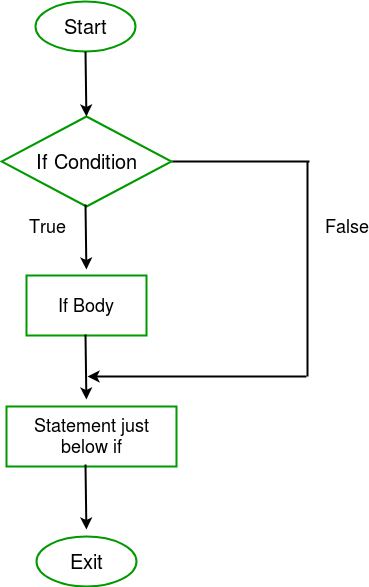Example :

## Perl

 `# Perl program to illustrate if statement`   `\$a` `= 10; `   `# if condition to check` `# for even number` `if``(``\$a` `% 2 == 0 )` `{ ` `    ``printf` `"Even Number"``; ` `} `

Output :

`Even Number`

if – else Statement

The if statement evaluates the code if the condition is true but what if the condition is not true, here comes the else statement. It tells the code what to do when the if condition is false.
Syntax :

```if(condition)
{
# code if condition is true
}
else
{
# code if condition is false
}  ```

Flowchart :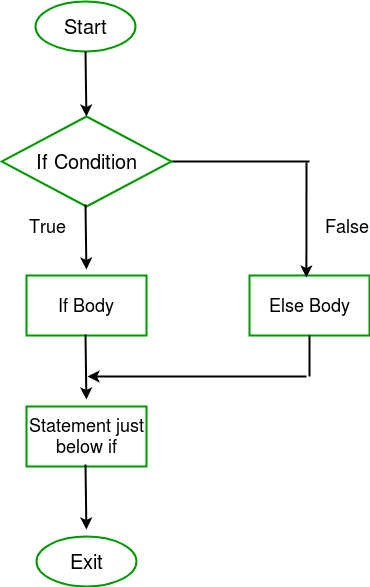Example :

## Perl

 `# Perl program to illustrate ` `# if - else statement`   `\$a` `= 21; `   `# if condition to check` `# for even number` `if``(``\$a` `% 2 == 0 )` `{ ` `    ``printf` `"Even Number"``; ` `} ` `else` `{   ` `    ``printf` `"Odd Number\n"``; ` `}`

Output :

`Odd Number`

Nested – if Statement

if statement inside an if statement is known as nested if. if statement in this case is the target of another if or else statement. When more than one condition needs to be true and one of the condition is the sub-condition of parent condition, nested if can be used.
Syntax :

```if (condition1)
{
# Executes when condition1 is true

if (condition2)
{
# Executes when condition2 is true
}
}```

Flowchart :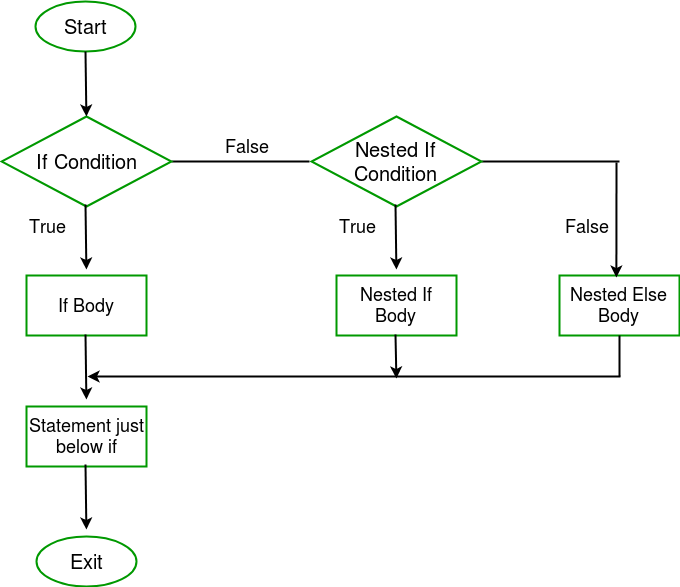Example :

## Perl

 `# Perl program to illustrate ` `# Nested if statement`   `\$a` `= 10;` `  `  `if``(``\$a` `% 2 ==0)` `{ ` `     ``# Nested - if statement` `     ``# Will only be executed ` `     ``# if above if statement` `     ``# is true` `    ``if``(``\$a` `% 5 == 0)` `    ``{  ` `         ``printf` `"Number is divisible by 2 and 5\n"``;  ` `     ``} ` `}`

Output :

`Number is divisible by 2 and 5`

If – elsif – else ladder Statement

Here, a user can decide among multiple options. The if statements are executed from the top down. As soon as one of the conditions controlling the if is true, the statement associated with that get executed, and the rest of the ladder is bypassed. If none of the conditions is true, then the final else statement will be executed.
Syntax :

```if(condition1)
{
# code to be executed if condition1 is true
}
elsif(condition2)
{
# code to be executed if condition2 is true
}
elsif(condition3)
{
# code to be executed if condition3 is true
}
...
else
{
# code to be executed if all the conditions are false
}  ```

Flowchart :

Example :

## Perl

 `# Perl program to illustrate ` `# if - elseif ladder statement`   `\$i` `= 20;  `   `if``(``\$i` `== 10)` `{` `    ``printf` `"i is 10\n"``;  ` `}`   `elsif``(``\$i` `== 15)` `{` `    ``printf` `"i is 15\n"``;` `}`   `elsif``(``\$i` `== 20)` `{` `    ``printf` `"i is 20\n"``;` `}`   `else` `{` `    ``printf` `"i is not present\n"``;` `}`

Output :

`i is 20`

unless Statement

In this case if the condition is false then the statements will execute. The number 0, the empty string “”, character ‘0’, the empty list (), and undef are all false in a boolean context and all other values are true.
Syntax :

```unless(boolean_expression)
{
# will execute if the given condition is false
}```

Flowchart :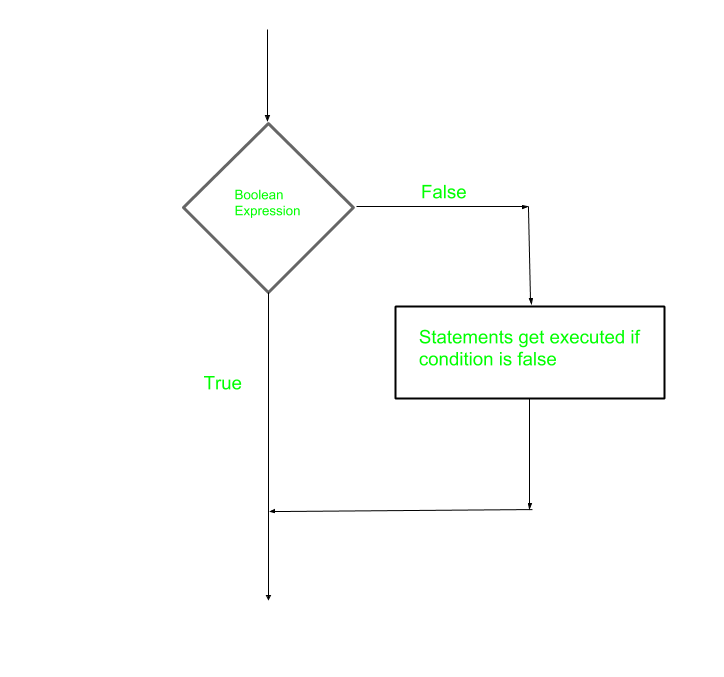Example :

## Perl

 `# Perl program to illustrate ` `# unless statement`   `\$a` `= 10;`   `unless``(``\$a` `!= 10)` `{`   `    ``# if condition is false then` `    ``# print the following` `    ``printf` `"a is not equal to 10\n"``;` `}`

Output :

`a is not equal to 10`

Unless-else Statement

Unless statement can be followed by an optional else statement, which executes when the boolean expression is true.
Syntax :

```unless(boolean_expression)
{
# execute if the given condition is false
}

else
{
# execute if the given condition is true
}```

Flowchart :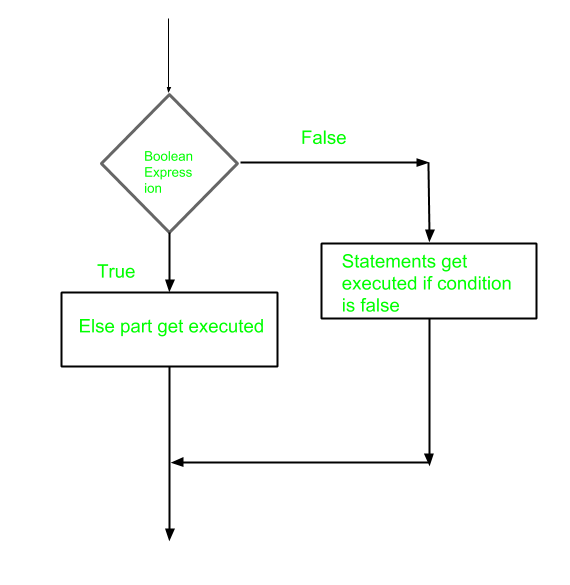Example :

## Perl

 `# Perl program to illustrate ` `# unless - else statement`   `\$a` `= 10;`   `unless``(``\$a` `== 10)` `{`   `    ``# if condition is false then` `    ``# print the following` `    ``printf` `"a is not equal to 10\n"``;` `}`   `else` `{ `   `    ``# if condition is true then` `    ``# print the following` `    ``printf` `"a is equal to 10\n"``;` `}`

Output :

`a is equal to 10`

Unless – elsif Statement

Unless statement can be followed by an optional elsif…else statement, which is very useful to test the various conditions using single unless…elsif statement.
Points to Remember :

• Unless statement can have zero to many elsif’s and all that must come before the else.
• Unless statement can have zero or one else’s and that must come after any elsif’s.
• Once an elsif succeeds, then none of remaining elsif’s or else’s will be tested.

Syntax :

```unless(boolean_expression 1)
{
# Executes when the boolean expression 1 is false
}
elsif( boolean_expression 2)
{
# Executes when the boolean expression 2 is true
}
else
{
# Executes when the none of the above condition is met
}```

Flowchart :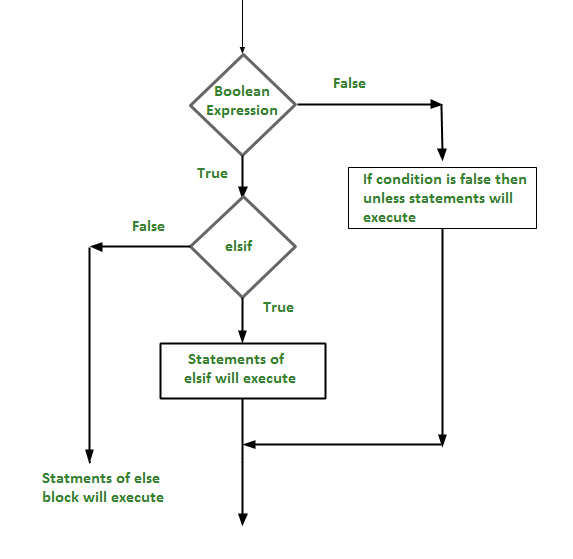Example :

## Perl

 `# Perl program to illustrate ` `# unless - elsif statement` `\$a` `= 50;`   `unless``(``\$a`  `==  60) ` `{`   `   ``# if condition is false` `   ``printf` `"a is not equal to 60\n"``;` `} ` `elsif``(``\$a` `==  60) ` `{   ` `   `  `   ``# if condition is true` `   ``printf` `"a is equal to 60\n"``;` `}` `else` `{  `   `   ``# if none of the condition matches` `   ``printf` `"The value of a is \$a\n"``;` `}`

Output

`a is not equal to 60`

Output :

`a is not equal to 60`

Whether you're preparing for your first job interview or aiming to upskill in this ever-evolving tech landscape, GeeksforGeeks Courses are your key to success. We provide top-quality content at affordable prices, all geared towards accelerating your growth in a time-bound manner. Join the millions we've already empowered, and we're here to do the same for you. Don't miss out - check it out now!